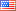# Revision history [back]

### Density plot aspect ratio

I am trying to make a very high aspect ratio density plot, but I can't seem to manually adjust the aspect ratio, and the automatically generated plot ends up being just a very pretty line. What am I doing wrong? The code I'm using is below. I don't know how to use Markdown to make it look pretty, sorry :(

print("Diffusion length data")

k = 1.381E-023

T = 300

q = 1.602E-019

var('ue')

De(ue) = uekT/q

var('tau')

Le = (tau10^-9De)^(1/2)

D = density_plot(Le*10^4, (tau, 0, 3), (ue, 0, 500), cmap="jet")

D.show(aspect_ratio=0.05)2 No.2 RevisionShashank1897 ●30 ●50 ●83

### Density plot aspect ratio

I am trying to make a very high aspect ratio density plot, but I can't seem to manually adjust the aspect ratio, and the automatically generated plot ends up being just a very pretty line. What am I doing wrong? The code I'm using is below. I don't know how to use Markdown to make it look pretty, sorry :(

print("Diffusion length data") data")
k = 1.381E-023 1.381E-023
T = 300 300
q = 1.602E-019 var('ue') 1.602E-019
var('ue')
De(ue) = uekT/q var('tau') ue*k*T/q
var('tau')
Le = (tau10^-9De)^(1/2) (tau*10^-9*De)^(1/2)
D = density_plot(Le*10^4, (tau, 0, 3), (ue, 0, 500), cmap="jet") D.show(aspect_ratio=0.05)cmap="jet")
D.show(aspect_ratio=0.05)